# An Even Easier Introduction to CUDA (Copied)

By Mark Harris | January 25, 2017 Tags: accelerated computing, Beginner, CUDA, Machine Learning and AI

This post is a super simple introduction to CUDA, the popular parallel computing platform and programming model from NVIDIA. I wrote a previous “Easy Introduction” to CUDA in 2013 that has been very popular over the years. But CUDA programming has gotten easier, and GPUs have gotten much faster, so it’s time for an updated (and even easier) introduction.

CUDA C++ is just one of the ways you can create massively parallel applications with CUDA. It lets you use the powerful C++ programming language to develop high performance algorithms accelerated by thousands of parallel threads running on GPUs. Many developers have accelerated their computation- and bandwidth-hungry applications this way, including the libraries and frameworks that underpin the ongoing revolution in artificial intelligence known as Deep Learning.

So, you’ve heard about CUDA and you are interested in learning how to use it in your own applications. If you are a C or C++ programmer, this blog post should give you a good start. To follow along, you’ll need a computer with an CUDA-capable GPU (Windows, Mac, or Linux, and any NVIDIA GPU should do), or a cloud instance with GPUs (AWS, Azure, IBM SoftLayer, and other cloud service providers have them). You’ll also need the free CUDA Toolkit installed.

Let’s get started!

## Starting Simple

We’ll start with a simple C++ program that adds the elements of two arrays with a million elements each.

``````1.#include <iostream>
2.#include <math.h>
3.
4.// function to add the elements of two arrays
5.void add(int n, float *x, float *y)
6.{
7.  for (int i = 0; i < n; i++)
8.      y[i] = x[i] + y[i];
9.}
10.
11.int main(void)
12.{
13.  int N = 1<<20; // 1M elements
14.
15.  float *x = new float[N];
16.  float *y = new float[N];
17.
18.  // initialize x and y arrays on the host
19.  for (int i = 0; i < N; i++) {
20.    x[i] = 1.0f;
21.    y[i] = 2.0f;
22.  }
23.
24.  // Run kernel on 1M elements on the CPU
26.
27.  // Check for errors (all values should be 3.0f)
28.  float maxError = 0.0f;
29.  for (int i = 0; i < N; i++)
30.    maxError = fmax(maxError, fabs(y[i]-3.0f));
31.  std::cout << "Max error: " << maxError << std::endl;
32.
33.  // Free memory
34.  delete [] x;
35.  delete [] y;
36.
37.  return 0;
38.}
``````

First, compile and run this C++ program. Put the code above in a file and save it as `add.cpp`, and then compile it with your C++ compiler. I’m on a Mac so I’m using `clang++`, but you can use `g++` on Linux or MSVC on Windows.

``````1.> clang++ add.cpp -o add
``````

Then run it:

``````1.> ./add
2. Max error: 0.000000
``````

(On Windows you may want to name the executable `add.exe` and run it with `.\add`.)

As expected, it prints that there was no error in the summation and then exits. Now I want to get this computation running (in parallel) on the many cores of a GPU. It’s actually pretty easy to take the first steps.

First, I just have to turn our add function into a function that the GPU can run, called a `kernel` in CUDA. To do this, all I have to do is add the specifier `__global__` to the function, which tells the CUDA C++ compiler that this is a function that runs on the GPU and can be called from CPU code.

``````1.// CUDA Kernel function to add the elements of two arrays on the GPU
2.__global__
3.void add(int n, float *x, float *y)
4.{
5.  for (int i = 0; i < n; i++)
6.      y[i] = x[i] + y[i];
7.}
``````

These `__global__` functions are known as kernels, and code that runs on the GPU is often called `device code`, while code that runs on the CPU is `host code`.

## Memory Allocation in CUDA

To compute on the GPU, I need to allocate memory accessible by the GPU. Unified Memory in CUDA makes this easy by providing a single memory space accessible by all GPUs and CPUs in your system. To allocate data in unified memory, call `cudaMallocManaged()`, which returns a pointer that you can access from host (CPU) code or device (GPU) code. To free the data, just pass the pointer to `cudaFree()`.

I just need to replace the calls to new in the code above with calls to `cudaMallocManaged()`, and replace calls to delete [] with calls to `cudaFree`.

``````1.  // Allocate Unified Memory -- accessible from CPU or GPU
2.  float *x, *y;
3.  cudaMallocManaged(&x, N*sizeof(float));
4.  cudaMallocManaged(&y, N*sizeof(float));
5.
6.  ...
7.
8.  // Free memory
9.  cudaFree(x);
10.  cudaFree(y);
``````

Finally, I need to launch the `add()` kernel, which invokes it on the GPU. CUDA kernel launches are specified using the `triple angle` bracket syntax `<<< >>>`. I just have to add it to the call to add before the parameter list.

``````1.add<<<1, 1>>>(N, x, y);
``````

Easy! I’ll get into the details of what goes inside the angle brackets soon; for now all you need to know is that this line launches one GPU thread to run `add()`.

Just one more thing: I need the CPU to wait until the kernel is done before it accesses the results (because CUDA kernel launches don’t block the calling CPU thread). To do this I just call `cudaDeviceSynchronize()` before doing the final error checking on the CPU.

Here’s the complete code:

``````1.#include <iostream>
2.#include <math.h>
3.// Kernel function to add the elements of two arrays
4.__global__
5.void add(int n, float *x, float *y)
6.{
7.  for (int i = 0; i < n; i++)
8.    y[i] = x[i] + y[i];
9.}
10.
11.int main(void)
12.{
13.  int N = 1<<20;
14.  float *x, *y;
15.
16.  // Allocate Unified Memory – accessible from CPU or GPU
17.  cudaMallocManaged(&x, N*sizeof(float));
18.  cudaMallocManaged(&y, N*sizeof(float));
19.
20.  // initialize x and y arrays on the host
21.  for (int i = 0; i < N; i++) {
22.    x[i] = 1.0f;
23.    y[i] = 2.0f;
24.  }
25.
26.  // Run kernel on 1M elements on the GPU
28.
29.  // Wait for GPU to finish before accessing on host
31.
32.  // Check for errors (all values should be 3.0f)
33.  float maxError = 0.0f;
34.  for (int i = 0; i < N; i++)
35.    maxError = fmax(maxError, fabs(y[i]-3.0f));
36.  std::cout << "Max error: " << maxError << std::endl;
37.
38.  // Free memory
39.  cudaFree(x);
40.  cudaFree(y);
41.
42.  return 0;
43.}
``````

CUDA files have the file extension `.cu`. So save this code in a file called `add.cu` and compile it with `nvcc`, the CUDA C++ compiler.

``````1.> nvcc add.cu -o add_cuda
3.Max error: 0.000000
``````

This is only a first step, because as written, this kernel is only correct for a single thread, since every thread that runs it will perform the add on the whole array. Moreover, there is a race condition since multiple parallel threads would both read and write the same locations.

Note: on Windows, you need to make sure you set Platform to `x64` in the Configuration Properties for your project in Microsoft Visual Studio.

## Profile it!

I think the simplest way to find out how long the kernel takes to run is to run it with `nvprof`, the command line GPU profiler that comes with the CUDA Toolkit. Just type `nvprof ./add_cuda` on the command line: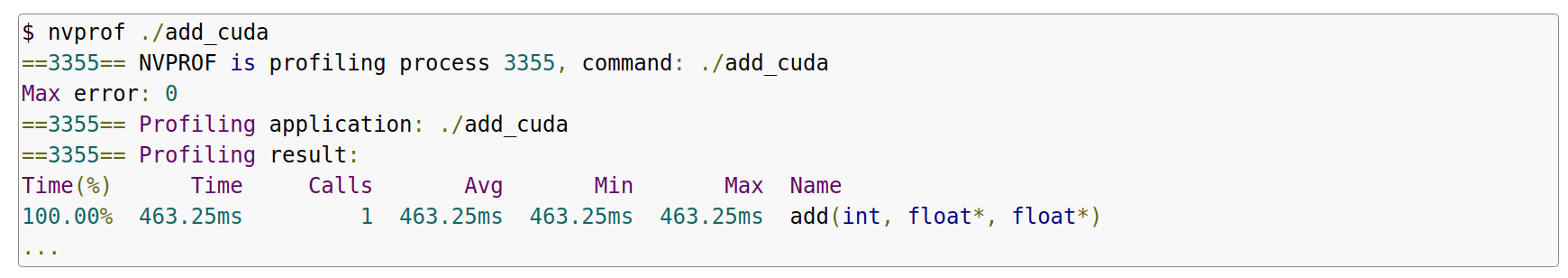Above is the truncated output from `nvprof`, showing a single call to add. It takes about half a second on an NVIDIA Tesla K80 accelerator, and about the same time on an NVIDIA GeForce GT 740M in my 3-year-old Macbook Pro.

Let’s make it faster with parallelism.

Now that you’ve run a kernel with one thread that does some computation, how do you make it parallel? The key is in CUDA’s `<<<1, 1>>>` syntax. This is called the `execution configuration`, and it tells the CUDA runtime how many parallel threads to use for the launch on the GPU. There are two parameters here, but let’s start by changing the second one: `the number of threads in a thread block`. CUDA GPUs run kernels using blocks of threads that are a multiple of `32` in size, so `256` threads is a reasonable size to choose.

``````1.add<<<1, 256>>>(N, x, y);
``````

If I run the code with only this change, it will do the computation once per thread, rather than spreading the computation across the parallel threads. To do it properly, I need to modify the kernel. CUDA C++ provides keywords that let kernels get the indices of the running threads. Specifically, `threadIdx.x` contains the index of the current thread within its block, and `blockDim.x` contains the number of threads in the block. I’ll just modify the loop to stride through the array with parallel threads.

``````1.__global__
2.void add(int n, float *x, float *y)
3.{
5.  int stride = blockDim.x;
6.  for (int i = index; i < n; i += stride)
7.      y[i] = x[i] + y[i];
8.}
``````

The `add` function hasn’t changed that much. In fact, setting index to 0 and stride to 1 makes it semantically identical to the first version.

Save the file as `add_block.cu` and compile and run it in `nvprof` again. For the remainder of the post I’ll just show the relevant line from the output.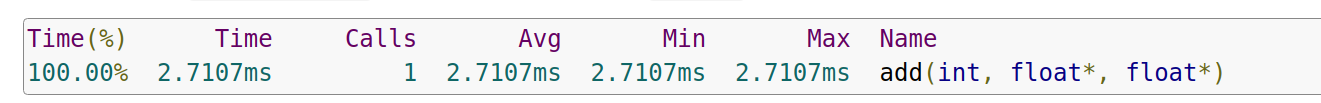That’s a big speedup (463ms down to 2.7ms), but not surprising since I went from 1 thread to 256 threads. The K80 is faster than my little Macbook Pro GPU (at 3.2ms). Let’s keep going to get even more performance.

## Out of the Blocks

• CUDA GPUs have many parallel processors grouped into `Streaming Multiprocessors`, or `SMs`.

• Each SM can run multiple concurrent thread blocks.

• As an example, a Tesla P100 GPU based on the Pascal GPU Architecture has `56` SMs, each capable of supporting up to `2048` active threads.

• To take full advantage of all these threads, we should launch the kernel with multiple thread blocks.

By now you may have guessed that the first parameter of the execution configuration specifies `the number of thread blocks`. Together, the blocks of parallel threads make up what is known as the `grid`. Since I have `N` elements to process, and `256` threads per block, I just need to calculate the number of blocks to get at least `N` threads. I simply divide `N` by the block size (being careful to round up in case `N` is not a multiple of `blockSize`).

``````1.int blockSize = 256;
2.int numBlocks = (N + blockSize - 1) / blockSize;
``````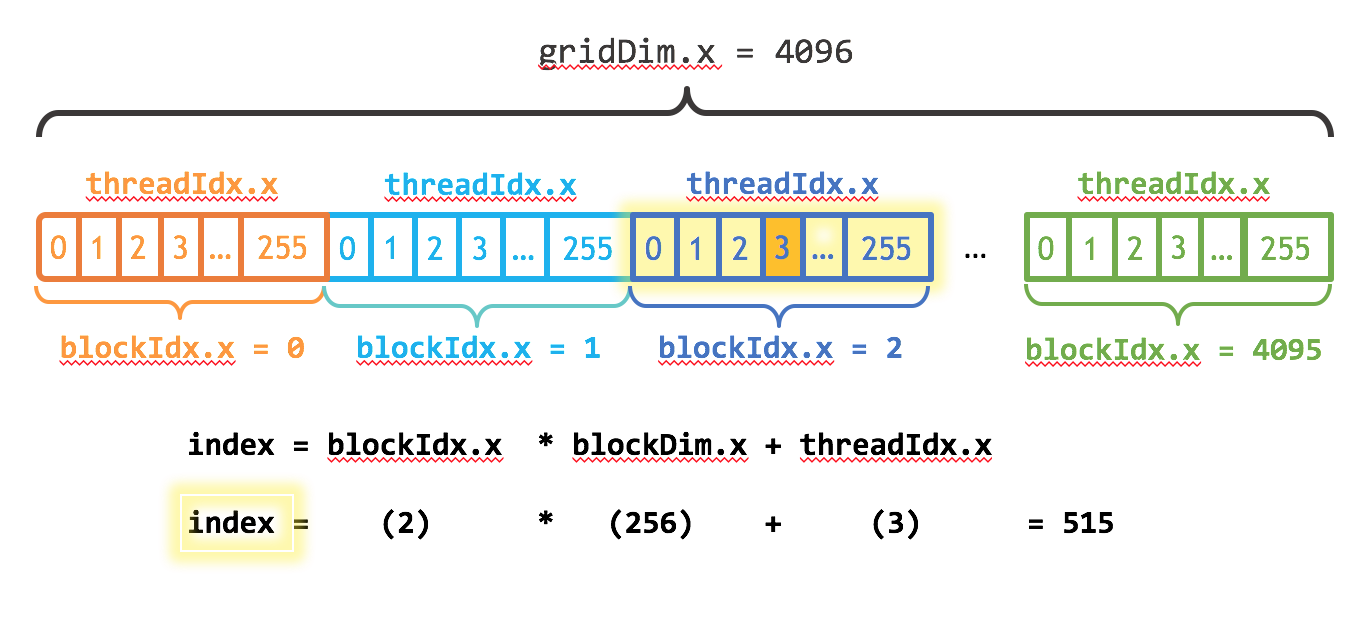I also need to update the kernel code to take into account the entire grid of thread blocks. CUDA provides `gridDim.x`, which contains the number of blocks in the grid, and `blockIdx.x`, which contains the index of the current thread block in the grid. Figure 1 illustrates the the approach to indexing into an array (one-dimensional) in CUDA using `blockDim.x`, `gridDim.x`, and `threadIdx.x`. The idea is that each thread gets its index by computing the offset to the beginning of its block (the block index times the block size: `blockIdx.x * blockDim.x`) and adding the thread’s index within the block (`threadIdx.x`). The code `blockIdx.x * blockDim.x + threadIdx.x` is idiomatic CUDA.

``````1.__global__
2.void add(int n, float *x, float *y)
3.{
4.  int index = blockIdx.x * blockDim.x + threadIdx.x;
5.  int stride = blockDim.x * gridDim.x;
6.  for (int i = index; i < n; i += stride)
7.    y[i] = x[i] + y[i];
8.}
``````

The updated kernel also sets `stride` to the total number of threads in the grid (`blockDim.x * gridDim.x`). This type of loop in a CUDA kernel is often called a `grid-stride` loop.

Save the file as `add_grid.cu` and compile and run it in `nvprof` again.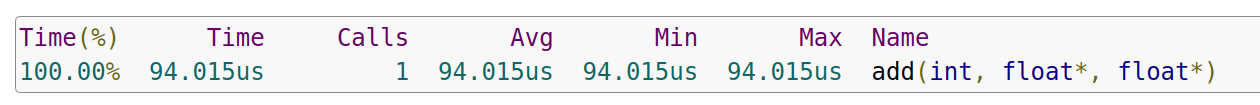That’s another 28x speedup, from running multiple blocks on all the SMs of a K80! We’re only using one of the 2 GPUs on the K80, but each GPU has 13 SMs. Note the GeForce in my laptop has 2 (weaker) SMs and it takes 680us to run the kernel.

## CUDA kernel and thread hierarchy

• Figure 1 shows that the CUDA kernel is a function that gets executed on GPU. The parallel portion of your applications is executed K times in parallel by K different CUDA threads, as opposed to only one time like regular C/C++ functions.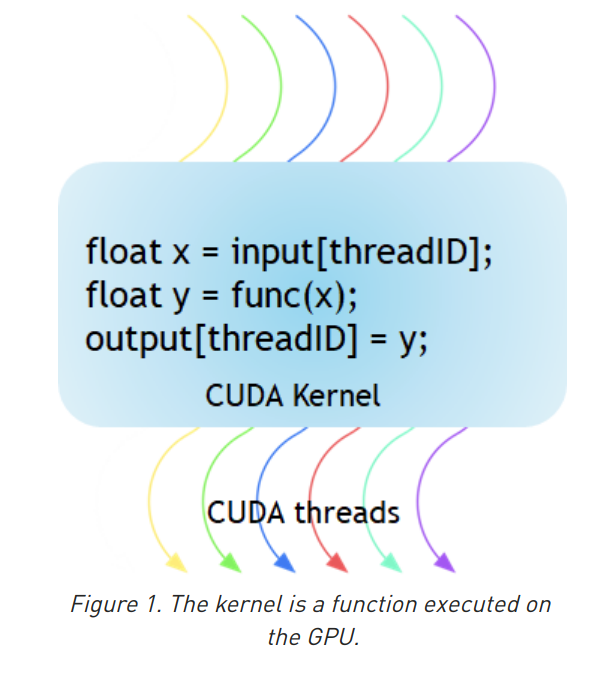• Every CUDA kernel starts with a `__global__` declaration specifier. Programmers provide a `unique global ID` to each thread by using built-in variables.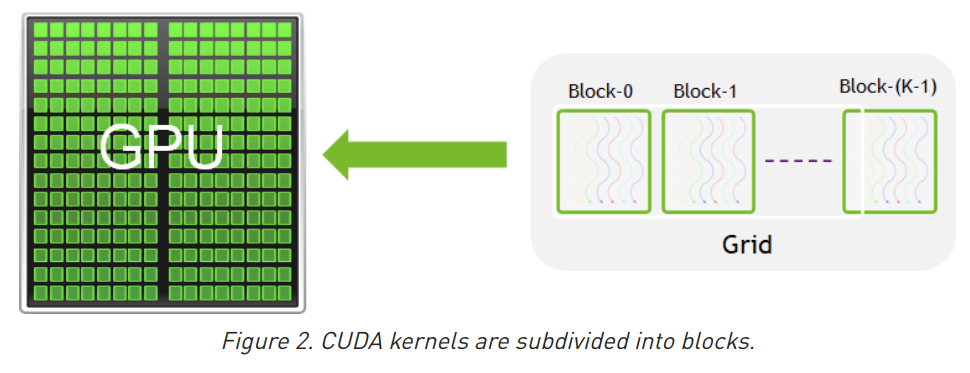• A group of threads is called a CUDA block. CUDA blocks are grouped into a grid.

• A kernel is executed as `a grid of blocks of threads` (Figure 2).

• Each CUDA block is executed by `one streaming multiprocessor (SM)` and cannot be migrated to other SMs in GPU (except during preemption, debugging, or CUDA dynamic parallelism).

• `One SM can run several concurrent CUDA blocks depending on the resources needed by CUDA blocks.`

• Recall: For example, a Tesla P100 GPU based on the Pascal GPU Architecture has `56` SMs, each capable of supporting up to `2048` active threads.

• Each kernel is executed on one device and CUDA supports running multiple kernels on a device at one time.

• Figure 3 shows the kernel execution and mapping on hardware resources available in GPU.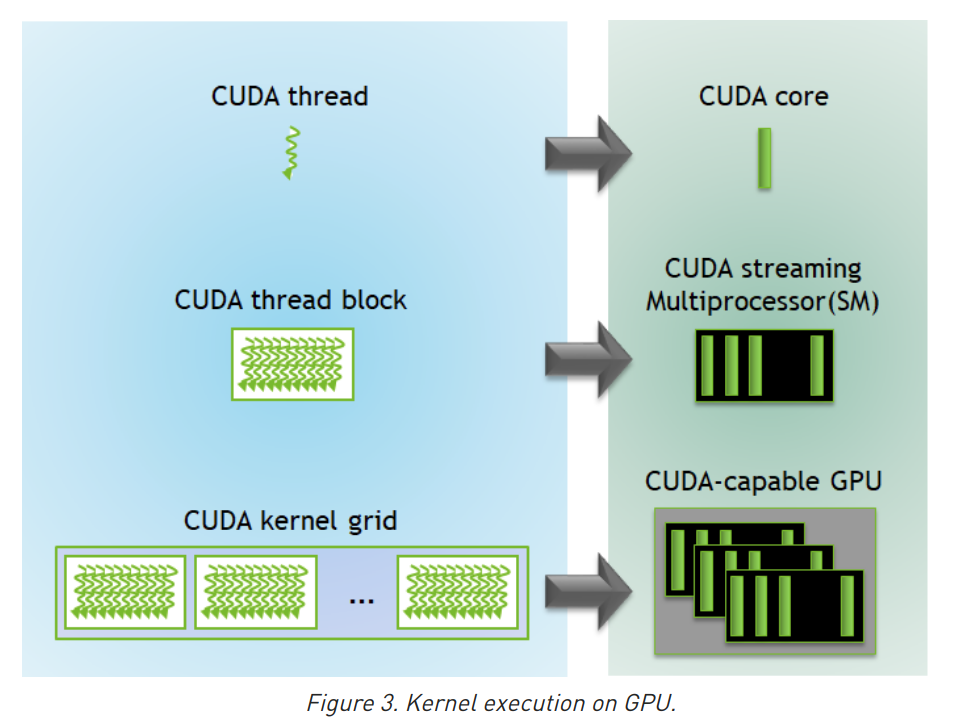CUDA defines built-in 3D variables for threads and blocks. Threads are indexed using the built-in 3D variable `threadIdx`. Three-dimensional indexing provides a natural way to index elements in `vectors`, `matrix`, and `volume` and makes CUDA programming easier. Similarly, blocks are also indexed using the in-built 3D variable called `blockIdx`.

Here are a few noticeable points:

• CUDA architecture limits the numbers of threads per block (`1024` threads per block limit).

• The dimension of the thread block is accessible within the kernel through the built-in `blockDim` variable.

• All threads within a block can be synchronized using an intrinsic function `__syncthreads`. With `__syncthreads`, all threads in the block must wait before anyone can proceed.

• The number of threads per block and the number of blocks per grid specified in the `<<<…>>>` syntax can be of type int or `dim3`. These triple angle brackets mark a call from host code to device code. It is also called a kernel launch.

The CUDA program for adding two matrices below shows multi-dimensional `blockIdx` and `threadIdx` and other variables like `blockDim`. In the example below, a 2D block is chosen for ease of indexing and each block has 256 threads with 16 each in x and y-direction. The total number of blocks are computed using the data size divided by the size of each block.

``````1.// Kernel - Adding two matrices MatA and MatB
2.__global__ void MatAdd(float MatA[N][N], float MatB[N][N],
3.float MatC[N][N])
4.{
5.    int i = blockIdx.x * blockDim.x + threadIdx.x;
6.    int j = blockIdx.y * blockDim.y + threadIdx.y;
7.    if (i < N && j < N)
8.        MatC[i][j] = MatA[i][j] + MatB[i][j];
9.}
10.
11.int main()
12.{
13.    ...
14.    // Matrix addition kernel launch from host code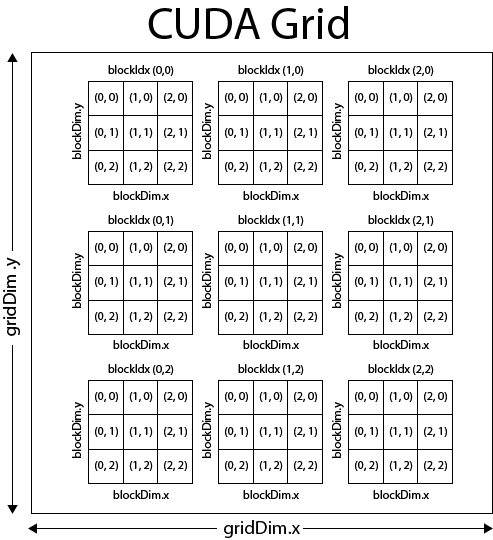In the image above we see that this example grid is divided into nine thread blocks (`3×3`), each thread block consists of 9 threads (`3×3`) for a total of 81 threads for the kernel grid.
This image only shows `2-dimensional` grid, but if the graphics device supports compute capability 2.0, then the grid of thread blocks can actually be partitioned into `1, 2 or 3 dimensions`, otherwise if the device supports compute capability 1.x, then thread blocks can be partitioned into 1, or 2 dimensions (in this case, then the 3rd dimension should always be set to 1).